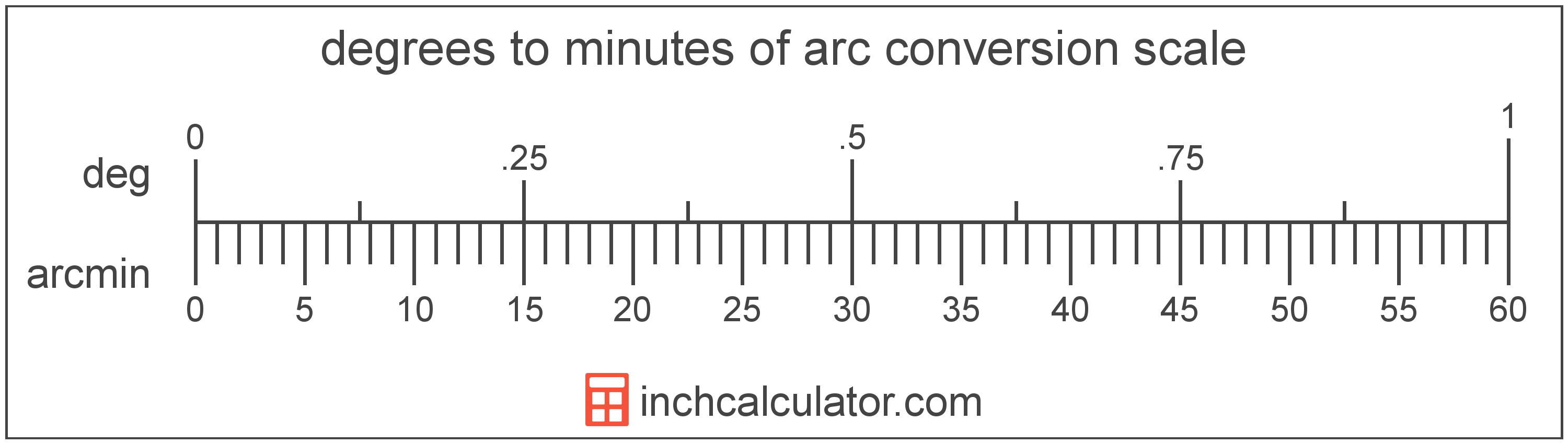# Degrees to Minutes of Arc Converter

Enter the angle in degrees below to get the value converted to minutes of arc.

Results in Minutes of Arc:1° = 60 arcmin

Do you want to convert minutes of arc to degrees?

## How to Convert Degrees to Minutes of Arc

To convert a measurement in degrees to a measurement in minutes of arc, multiply the angle by the following conversion ratio: 60 minutes of arc/degree.

Since one degree is equal to 60 minutes of arc, you can use this simple formula to convert:

minutes of arc = degrees × 60

The angle in minutes of arc is equal to the angle in degrees multiplied by 60.

For example, here's how to convert 5 degrees to minutes of arc using the formula above.
minutes of arc = (5° × 60) = 300'### How Many Minutes of Arc Are in a Degree?

There are 60 minutes of arc in a degree, which is why we use this value in the formula above.

1° = 60'

### Uses for Degrees, Minutes, & Seconds

For measurements in cartography and astronomy, degrees are often expressed in decimal form or degrees, minutes, and seconds (sometimes called DMS). Minutes and seconds are used to express portions of angle smaller than one degree.

You can use our decimal to DMS calculator to convert an angle to degrees, minutes, & seconds form.

## What is a Degree?

A degree is a measure of angle equal to 1/360th of a revolution, or circle. The number 360 has 24 divisors, making it a fairly easy number to work with. There are also 360 days in the Persian calendar year, and many theorize that early astronomers used 1 degree per day.

The degree is an SI accepted unit for angle for use with the metric system. A degree is sometimes also referred to as a degree of arc, arc degree, or arcdegree. Degrees can be abbreviated as °, and are also sometimes abbreviated as deg. For example, 1 degree can be written as 1° or 1 deg.

Degrees can also be expressed using arcminutes and arcseconds as an alternative to using the decimal form. Arcminutes and arcseconds are expressed using the prime (′) and double-prime (″) characters, respectively, although a single-quote and double-quote are often used for convenience.

One arcminute is equal to 1/60th of a degree, and one arcsecond is equal to 1/60th of an arcminute.

Protractors are commonly used to measure angles in degrees. They are semi-circle or full-circle devices with degree markings allowing a user to measure an angle in degrees. Learn more about how to use a protractor or download a printable protractor.

## What is a Minute of Arc?

The minute of arc is a unit of angle equal to 1/60th of one degree, or 1/21,600 of a circle. The minute of arc is also equal to π/10,800 radians.

A minute of arc is sometimes also referred to as an arc minute, arcminute, or minute arc. Minutes of arc can be abbreviated as arcmin, and are also sometimes abbreviated as MOA or amin. For example, 1 minute of arc can be written as 1 arcmin, 1 MOA, or 1 amin.

The minute of arc is most commonly represented using the prime (′), although the single-quote is commonly used. For instance, 1 minute of is most commonly expressed as 1′.

## Degree to Minute of Arc Conversion Table

Table showing various degree measurements converted to minutes of arc.
Degrees Minutes Of Arc
60'
120'
180'
240'
300'
360'
420'
480'
540'
10° 600'
11° 660'
12° 720'
13° 780'
14° 840'
15° 900'
16° 960'
17° 1,020'
18° 1,080'
19° 1,140'
20° 1,200'
21° 1,260'
22° 1,320'
23° 1,380'
24° 1,440'
25° 1,500'
26° 1,560'
27° 1,620'
28° 1,680'
29° 1,740'
30° 1,800'
31° 1,860'
32° 1,920'
33° 1,980'
34° 2,040'
35° 2,100'
36° 2,160'
37° 2,220'
38° 2,280'
39° 2,340'
40° 2,400'

## References

1. Collins Dictionary, Definition of 'degree', https://www.collinsdictionary.com/us/dictionary/english/degree